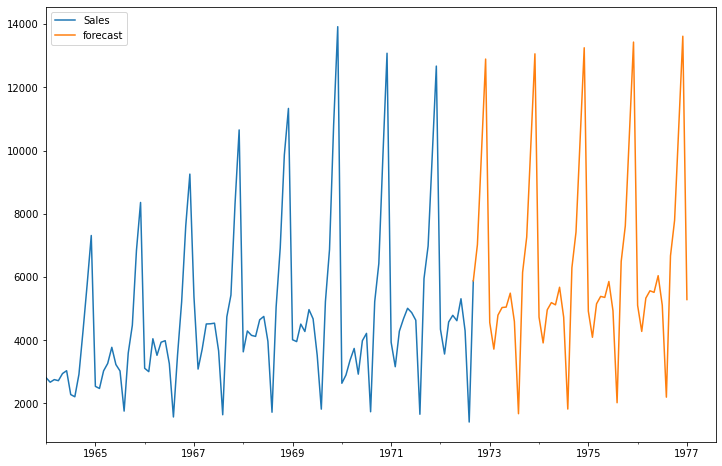# Time Series Analysis Using ARIMA From Statsmodels

ARIMA and exponential Moving averages are two methods for forecasting based on time series data. In this notebook, I will talk about ARIMA which is an acronym for Autoregressive Integrated Moving Averages.

## Autoregressive Integrated Moving Averages (ARIMA)

The general process for ARIMA models is the following:

• Visualize the Time Series Data
• Make the time series data stationary
• Plot the Correlation and AutoCorrelation Charts
• Construct the ARIMA Model or Seasonal ARIMA based on the data
• Use the model to make predictions

Let's go through these steps!

## Monthly Champagne Sales Data

In :
import numpy as np
import pandas as pd

import matplotlib.pyplot as plt
%matplotlib inline


For this example, I took the sales data which is available on kaggle https://www.kaggle.com/anupamshah/perrin-freres-monthly-champagne-sales

In :
df=pd.read_csv('perrin-freres-monthly-champagne-.csv')

In :
df.head()

Out:
Month Perrin Freres monthly champagne sales millions ?64-?72
0 1964-01 2815.0
1 1964-02 2672.0
2 1964-03 2755.0
3 1964-04 2721.0
4 1964-05 2946.0
In :
df.tail()

Out:
Month Perrin Freres monthly champagne sales millions ?64-?72
102 1972-07 4298.0
103 1972-08 1413.0
104 1972-09 5877.0
105 NaN NaN
106 Perrin Freres monthly champagne sales millions... NaN

## Data Cleaning

In :
## Cleaning up the data
df.columns=["Month","Sales"]

Out:
Month Sales
0 1964-01 2815.0
1 1964-02 2672.0
2 1964-03 2755.0
3 1964-04 2721.0
4 1964-05 2946.0

Our objective is to forecast the champagne sales.

In :
## Drop last 2 rows
df.drop(106,axis=0,inplace=True)


In :
df.tail()

Out:
Month Sales
101 1972-06 5312.0
102 1972-07 4298.0
103 1972-08 1413.0
104 1972-09 5877.0
105 NaN NaN
In :
df.drop(105,axis=0,inplace=True)

In :
df.tail()

Out:
Month Sales
100 1972-05 4618.0
101 1972-06 5312.0
102 1972-07 4298.0
103 1972-08 1413.0
104 1972-09 5877.0
In :
# Convert Month into Datetime
df['Month']=pd.to_datetime(df['Month'])

In :
df.head()

Out:
Month Sales
0 1964-01-01 2815.0
1 1964-02-01 2672.0
2 1964-03-01 2755.0
3 1964-04-01 2721.0
4 1964-05-01 2946.0
In :
df.set_index('Month',inplace=True)

In :
df.head()

Out:
Sales
Month
1964-01-01 2815.0
1964-02-01 2672.0
1964-03-01 2755.0
1964-04-01 2721.0
1964-05-01 2946.0
In :
df.describe()

Out:
Sales
count 105.000000
mean 4761.152381
std 2553.502601
min 1413.000000
25% 3113.000000
50% 4217.000000
75% 5221.000000
max 13916.000000

## Visualize the Time Series Data

In :
df.plot()

Out:
<AxesSubplot:xlabel='Month'>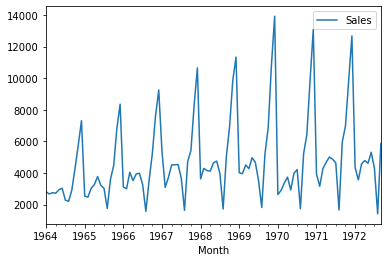## Testing For Stationarity of Data using Statsmodels adfuller

Stationary data means data which has no trend with respect to the time.

In :
### Testing For Stationarity

In :
test_result=adfuller(df['Sales'])

In :
#Ho: It is non stationary
#H1: It is stationary

labels = ['ADF Test Statistic','p-value','#Lags Used','Number of Observations Used']
for value,label in zip(result,labels):
print(label+' : '+str(value) )
if result <= 0.05:
print("P value is less than 0.05 that means we can reject the null hypothesis(Ho). Therefore we can conclude that data has no unit root and is stationary")
else:
print("Weak evidence against null hypothesis that means time series has a unit root which indicates that it is non-stationary ")

In :
adfuller_test(df['Sales'])

ADF Test Statistic : -1.8335930563276217
p-value : 0.3639157716602457
#Lags Used : 11
Number of Observations Used : 93
Weak evidence against null hypothesis that means time series has a unit root which indicates that it is non-stationary


## Differencing

Differencing helps remove the changes from the data and make data stationary.

In :
df['Sales First Difference'] = df['Sales'] - df['Sales'].shift(1)

In :
df['Sales'].shift(1)

Out:
Month
1964-01-01       NaN
1964-02-01    2815.0
1964-03-01    2672.0
1964-04-01    2755.0
1964-05-01    2721.0
...
1972-05-01    4788.0
1972-06-01    4618.0
1972-07-01    5312.0
1972-08-01    4298.0
1972-09-01    1413.0
Name: Sales, Length: 105, dtype: float64

we have monthly data so let us try a shift value of 12.

In :
df['Seasonal First Difference']=df['Sales']-df['Sales'].shift(12)

In :
df.head(14)

Out:
Sales Sales First Difference Seasonal First Difference
Month
1964-01-01 2815.0 NaN NaN
1964-02-01 2672.0 -143.0 NaN
1964-03-01 2755.0 83.0 NaN
1964-04-01 2721.0 -34.0 NaN
1964-05-01 2946.0 225.0 NaN
1964-06-01 3036.0 90.0 NaN
1964-07-01 2282.0 -754.0 NaN
1964-08-01 2212.0 -70.0 NaN
1964-09-01 2922.0 710.0 NaN
1964-10-01 4301.0 1379.0 NaN
1964-11-01 5764.0 1463.0 NaN
1964-12-01 7312.0 1548.0 NaN
1965-01-01 2541.0 -4771.0 -274.0
1965-02-01 2475.0 -66.0 -197.0

Let us check if the data now is stationary.

In :
## Again test dickey fuller test

ADF Test Statistic : -7.626619157213163
p-value : 2.060579696813685e-11
#Lags Used : 0
Number of Observations Used : 92
P value is less than 0.05 that means we can reject the null hypothesis(Ho). Therefore we can conclude that data has no unit root and is stationary

In :
df['Seasonal First Difference'].plot()

Out:
<AxesSubplot:xlabel='Month'>## Auto Regressive Model

In :
from statsmodels.tsa.arima_model import ARIMA
import statsmodels.api as sm

1. Partial Auto Correlation Function - Takes in to account the impact of direct variables only
2. Auto Correlation Function - Takes in to account the impact of all the variables (direct + indirect)

Let us plot lags on the horizontal and the correlations on vertical axis using plot_acf and plot_pacf function.

In :
from statsmodels.graphics.tsaplots import plot_acf,plot_pacf

In :
fig = plt.figure(figsize=(12,8))
fig = sm.graphics.tsa.plot_acf(df['Seasonal First Difference'].iloc[13:],lags=40,ax=ax1)
fig = sm.graphics.tsa.plot_pacf(df['Seasonal First Difference'].iloc[13:],lags=40,ax=ax2)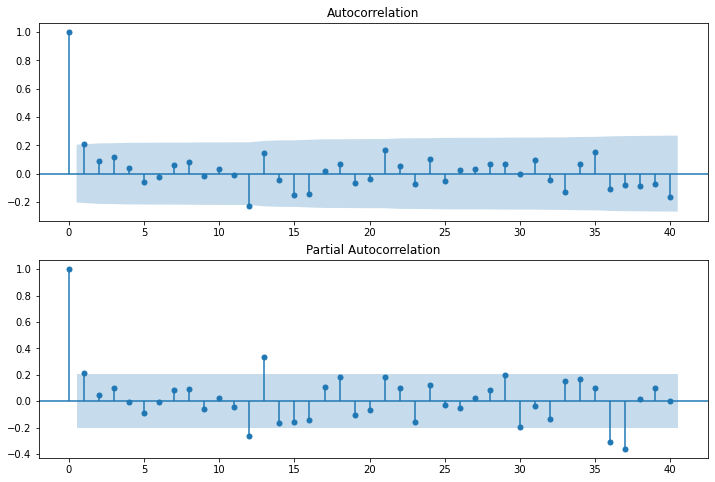In the graphs above, each spike(lag) which is above the dashed area considers to be statistically significant.

In [ ]:
# For non-seasonal data
#p=1 (AR specification), d=1 (Integration order), q=0 or 1 (MA specification/polynomial)
AR specification, Integration order, MA specification
from statsmodels.tsa.arima_model import ARIMA

In :
model=ARIMA(df['Sales'],order=(1,1,1))
model_fit=model.fit()

In :
model_fit.summary()

Out:
Dep. Variable: No. Observations: D.Sales 104 ARIMA(1, 1, 1) -951.126 css-mle 2227.262 Mon, 19 Apr 2021 1910.251 23:29:19 1920.829 02-01-1964 1914.536 - 09-01-1972
coef std err z P>|z| [0.025 0.975] 22.7835 12.405 1.837 0.066 -1.530 47.097 0.4343 0.089 4.866 0.000 0.259 0.609 -1.0000 0.026 -38.503 0.000 -1.051 -0.949
 Real Imaginary Modulus Frequency 2.3023 +0.0000j 2.3023 0.0000 1.0000 +0.0000j 1.0000 0.0000

We can also do line and density plot of residuals.

In :
from matplotlib import pyplot
residuals = pd.DataFrame(model_fit.resid)
residuals.plot()
pyplot.show()
# density plot of residuals
residuals.plot(kind='kde')
pyplot.show()
# summary stats of residuals
print(residuals.describe())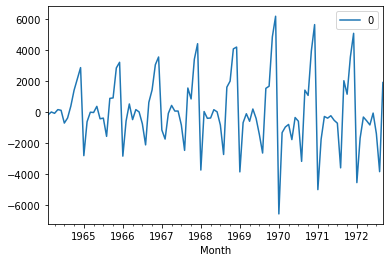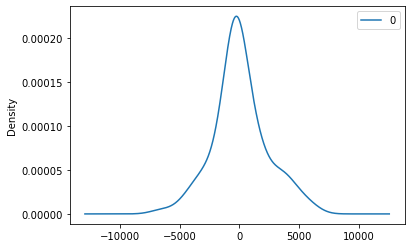0
count   104.000000
mean     87.809661
std    2257.896169
min   -6548.758563
25%    -821.138569
50%     -87.526059
75%    1221.542864
max    6177.251803


As we see above, mean is not exactly zero that means there is some bias in the data.

In :
df['forecast']=model_fit.predict(start=90,end=103,dynamic=True)
df[['Sales','forecast']].plot(figsize=(12,8))

Out:
<AxesSubplot:xlabel='Month'>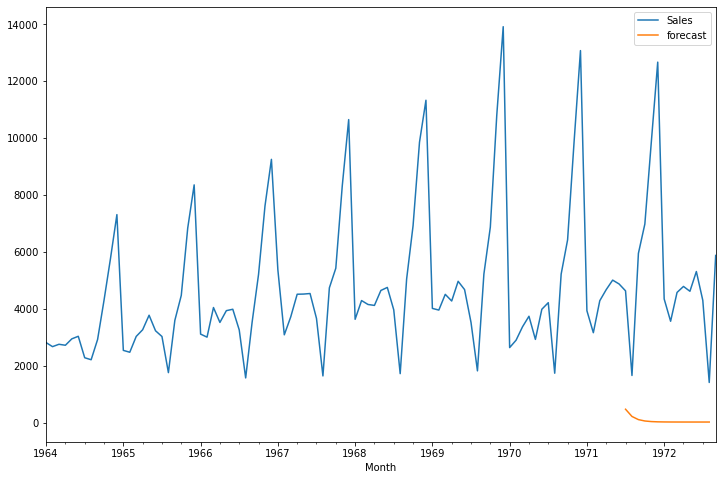If you observe the above, we are not getting good results using ARIMA because our data has seasonal behaviour, So let us try using seasonal ARIMA.

In :
import statsmodels.api as sm

In :
model=sm.tsa.statespace.SARIMAX(df['Sales'],order=(1, 1, 1),seasonal_order=(1,1,1,12))
results=model.fit()


Note above the seasonal_order tuples which takes the following format (Seasonal AR specification, Seasonal Integration order, Seasonal MA, Seasonal periodicity)

In :
results.summary()

Out:
Dep. Variable: No. Observations: Sales 105 SARIMAX(1, 1, 1)x(1, 1, 1, 12) -738.402 Mon, 19 Apr 2021 1486.804 23:29:33 1499.413 01-01-1964 1491.893 - 09-01-1972 opg
coef std err z P>|z| [0.025 0.975] 0.2790 0.081 3.433 0.001 0.120 0.438 -0.9494 0.043 -22.334 0.000 -1.033 -0.866 -0.4544 0.303 -1.499 0.134 -1.049 0.140 0.2450 0.311 0.788 0.431 -0.365 0.855 5.055e+05 6.12e+04 8.265 0.000 3.86e+05 6.25e+05
 Ljung-Box (L1) (Q): Jarque-Bera (JB): 0.26 8.7 0.61 0.01 1.18 -0.21 0.64 4.45

Warnings:
 Covariance matrix calculated using the outer product of gradients (complex-step).

Let us plot again line and density chart of residuals.

In :
from matplotlib import pyplot
residuals = pd.DataFrame(results.resid)
residuals.plot()
pyplot.show()
# density plot of residuals
residuals.plot(kind='kde')
pyplot.show()
# summary stats of residuals
print(residuals.describe())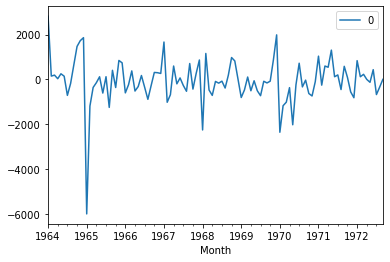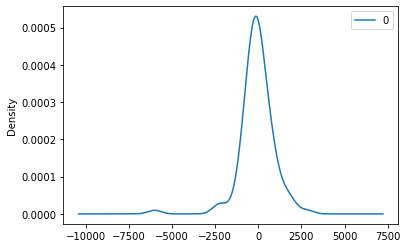0
count   105.000000
mean    -69.284285
std     996.587108
min   -6006.398653
25%    -475.852083
50%     -83.470336
75%     306.809583
max    2815.000000

In :
df['forecast']=results.predict(start=90,end=103,dynamic=True)
df[['Sales','forecast']].plot(figsize=(12,8))

Out:
<AxesSubplot:xlabel='Month'>Conclusion: If you compare the ARIMA and SARIMA results, SARIMA gives good result comapre to ARIMA.

## Forecasting for Next 5 years using SARIMA

In :
5*12

Out:
60
In :
from pandas.tseries.offsets import DateOffset
future_dates=[df.index[-1]+ DateOffset(months=x)for x in range(0,60)]

In :
future_datest_df=pd.DataFrame(index=future_dates[1:],columns=df.columns)

In :
future_datest_df.tail()

Out:
Sales Sales First Difference Seasonal First Difference forecast
1977-04-01 NaN NaN NaN NaN
1977-05-01 NaN NaN NaN NaN
1977-06-01 NaN NaN NaN NaN
1977-07-01 NaN NaN NaN NaN
1977-08-01 NaN NaN NaN NaN
In :
future_df=pd.concat([df,future_datest_df])

In :
future_df['forecast'] = results.predict(start = 104, end = 156, dynamic= True)
future_df[['Sales', 'forecast']].plot(figsize=(12, 8))

Out:
<AxesSubplot:>